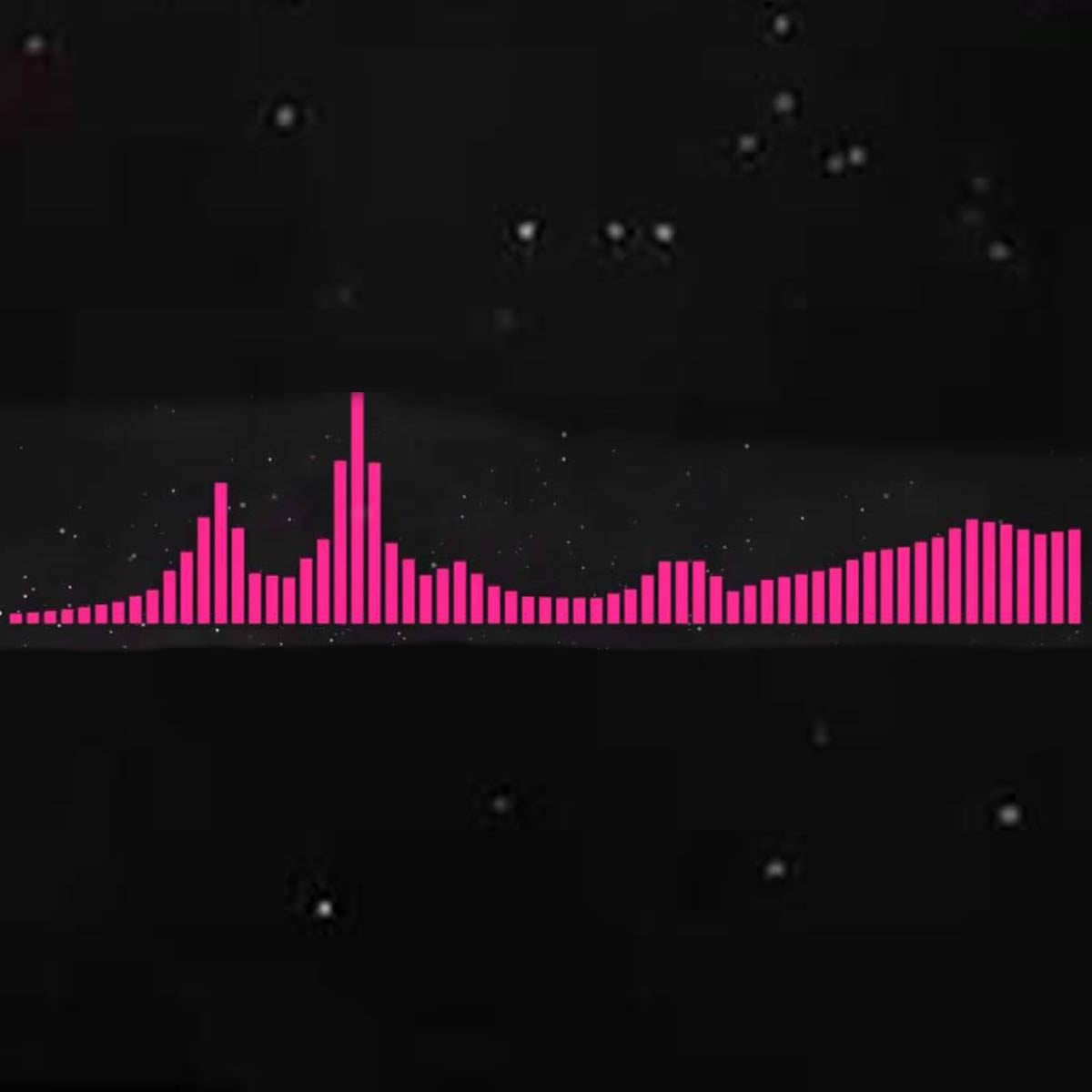# To The Stars

10 September 2020
3
• ##### RATING
5
(1)

i i i o O s P P O P s P O
i i i o O i s P O P s P O
g g g h H c c c Z l | l J l v v v x V c
g g g h H c c c Z l | H J l J l J H h H g
g g g h H c c c Z l | l J l v v v x V c
g g g h H c c c Z l | H J l J l J H h H g
s O i t w q

Rate This Music Sheet:

Average rating 5 / 5. Vote count: 1

No votes so far! Be the first to rate this music sheet.

Thank you for rating this song!

If you have any specific feedback about how to improve this music sheet, please submit this in the box below.

To The Stars is a song by Braken. Use your computer keyboard to play To The Stars music sheet on Virtual Piano. This is an Easy song and requires practice. The song To The Stars is classified in the genres: Dance, Electronica on Virtual Piano. You can also find other similar songs using EDM.

## Related

•7 7 7 6 7 9 7 \$
6 7 7 7 6 7 q 0
9 6 7 7 7 6 7 9
7 \$ 6 7 7 7 6 7
q 0 9 6 7 7 7 6
7 9 7 \$ 6 7 7 7
6 7 q 0 9 6 7 7
7 6 7 9 7 \$ 6 7
7 7 6 7 r r r e
r y r Q e r r r
e r i u y e r r
r e r y r Q e r
r r e r i u y e
r r r e r y r Q
e r r r e r i u
y e r r r e r y
r Q e r r r e r
7 * 9 0 Q 7 9 e
W Q 0 Q 7 * 9 0
Q 7 9 e W e r W
Q 7 * 9 0 Q 7 9
e W Q 0 Q 7 * 9
0 Q 7 9 e r e W
Q 9 * 7 6 7 7 \$
6 7 * 6 7 9 * 7
6 7 7 \$ * 9 * 6
3 y T r e r r Q
e r T e r y T r
6 9 Q 0 ( 7 e W
Q 9 * 7 6 7 6 7
6 7 6 7 7 * 9 0
Q 9 9 0 q 0 9 *
9 0 Q Q r 7 * 9
0 9 * e w Q 9 9
0 q 0 9 * 9 0 Q
Q r 7 * 9 5 \$ 4
9 ^ 7

Level: 3
Length: 02:46
Easy

#### Yugo Kanno

•[Eiv] b zlzl[tov] b zlzl[ypv] b zlzlc x z l
[Eiv] b zlzl[tov] b zlzl[yph] j l c xcxzl j
[Eiv] b zlzl[tov] b zlzl[ypv] b zlzlc [x c x z] l

Level: 4
Easy

#### Hatsune Miku

•[tsq]| jh s| | [ts0]| jh [da][yw]||d s [ytse]| jh s||f [tqf9]sdfsdfsd[tfe8]sd [sq] t [oj]htsq t o t [s0] t [oj]ht[da]w y o yf h [ha0] r [oj]hrse u t f [ytqf]sdfsd[ywf]sdfsd [tsq]| jh s| | [ts0]| jh [da][yw]||d s [ytse]| jh s||f [tqf9]sdfsdfsd[tfe8]sd [sq] t [oj]htsq t o t [s0] t [oj]ht[da]w y o yf h [ha0] r [oj]hrse u t f [ytqf]sdfsd[ywf]sdfsd 8| s h [g60] f d s [wrd] d s [ed9] d|| | df g[e9] f d s s||| [tqh9] f d [wt0] f s s [tqje] h d [ywr]||s [tqh9] f d [wut] f s s [tjie] h d [ywr] s h h [tqi]| j h [yw]| f d [ywro]| d fg[tie] f d s| [tsqi]| j h [ut0]| f d s| jh s
Level: 6
Length: 01:09
Intermediate